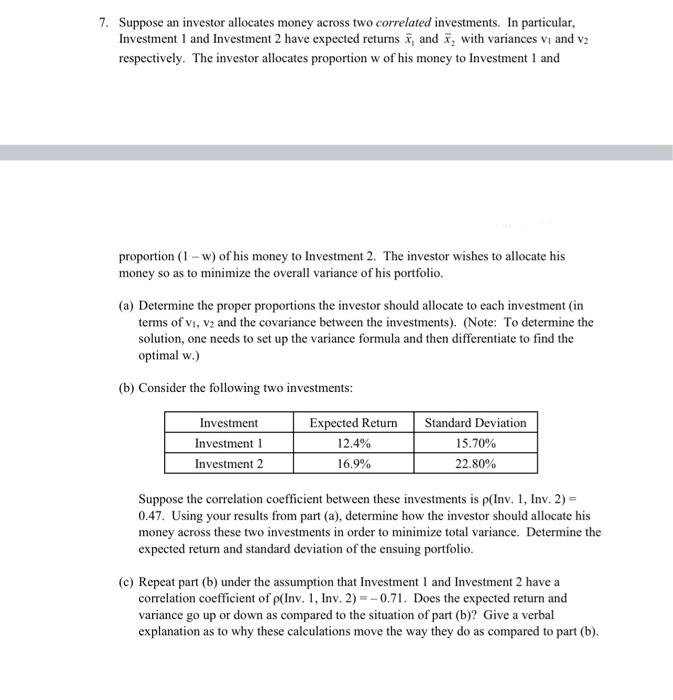Home / Expert Answers / Statistics and Probability / 7-suppose-an-investor-allocates-money-across-two-correlated-investments-in-particular-investment-pa606

# (Solved): 7. Suppose an investor allocates money across two correlated investments. In particular, Investment ...7. Suppose an investor allocates money across two correlated investments. In particular, Investment 1 and Investment 2 have expected returns and with variances and respectively. The investor allocates proportion of his money to Investment 1 and proportion of his money to Investment 2 . The investor wishes to allocate his money so as to minimize the overall variance of his portfolio. (a) Determine the proper proportions the investor should allocate to each investment (in terms of and the covariance between the investments). (Note: To determine the solution, one needs to set up the variance formula and then differentiate to find the optimal w.) (b) Consider the following two investments: Suppose the correlation coefficient between these investments is (Inv, 1, Inv, 2) = 0.47. Using your results from part (a), determine how the investor should allocate his money across these two investments in order to minimize total variance. Determine the expected return and standard deviation of the ensuing portfolio. (c) Repeat part (b) under the assumption that Investment 1 and Investment 2 have a correlation coefficient of (Inv. 1, Inv, 2. Does the expected return and variance go up or down as compared to the situation of part (b)? Give a verbal explanation as to why these calculations move the way they do as compared to part (b).

We have an Answer from Expert